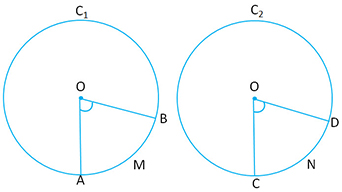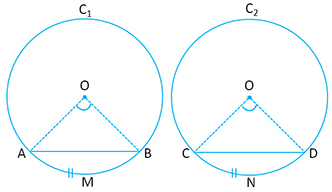Arc and Chord Properties:

Theorem 14: In equal circles, if two arcs subtends equal angles at the center, then the arcs are equal.Given: Two circles$C_1 \ and \ C_2$ with centers$O \ and \ P$ respectively.

Two$\widehat{AMB} \ and \ \widehat{CND}$ subtend equal angles$\angle AOB \ and\ \angle CPD$ at the respective centers.

To Prove:$\widehat{AMB} = \widehat{CND}$

Proof:  Join$AB \ and \ CD$

Now consider$\triangle AOB \ and\ \triangle CPD$$OA = OB$ (radius of the same circle)$CP = PD$ (radius of the same circle)

Now since the two circles are equal, it means that their radius are equal.

Hence$OA = PC \ and \ OB = PD$

Also given that$\angle AOB = \angle CPD$

Hence$\triangle AOB \cong \triangle CPD$ (S.A.S Postulate)

Therefore Now, if you place the two circles on the top of each other, you will see that they coincide and hence$\widehat{AMB} = \widehat{CND}$.

Hence Proved.Note: In the same triangle if$\widehat{AMB} \ and \ \widehat{CND}$ subtend equal angles at the center, then also the arcs are equal. For example:$\angle AOB = \angle COD \Rightarrow \widehat{AMB} =\widehat{CND}$

Theorem 15: In equal circles, if the two arcs are equal, they would subtend equal angles at the center.Given: Two circles$C_1 \ and \ C_2$ with centers$O \ and \ P$ respectively.$\widehat{AMB} = \widehat{CND}$ subtend$\angle AOB \ and\ \angle CPD$ at the respective centers.

To Prove:$\angle AOB = \angle CPD$

Proof:$OA = PC$ (radius of equal circles)$\widehat{AMB} = \widehat{CND}$

Therefore, if you were to place the circles$C_1 \ and \ C_2$ over each other, they will coincide. This also makes that$\angle AOB = \angle CPD$.

Hence Proved.

Theorem 16: In equal circles, if two chords are equal, they will cut equal arcs.Given: Two circles$C_1 \ and \ C_2$ with centers$O \ and \ P$ respectively. Also$Chord \ AB = Chord \ CD$

To Prove:$\widehat{AMB}=\widehat{CND}$

Proof: Consider$\triangle OAB \ and \ \triangle PCD$$OA = PC$ (radius of equal circles)$OB=PD$ (radius of equal circles)$AB = CD$ (Given)

Therefore$\triangle OAB \cong \triangle PCD$ (S.S.S postulate)$\Rightarrow \angle AOB = \angle CPD$

Therefore$\widehat{AMB}=\widehat{CND}$ ( In equal circles, if two arcs subtends equal angles at the center, then the arcs are equal.)

Hence Proved.

Theorem 17: In two equal circles, if the two arcs are equal the chords of the arcs are also equal. (Converse of Theorem 16)Given: Two circles$C_1 \ and \ C_2$ with centers$O \ and \ P$ respectively. Also$\widehat{AMB}=\widehat{CND}$

To Prove:$Chord \ AB = Chord \ CD$

Proof: Since$\widehat{AMB}=\widehat{CND}$$\angle AOB = \angle CPD$ (In equal circles, if the two arcs are equal, they would subtend equal angles at the center)

Consider$\triangle AOB \ and \ \triangle CPD$$\angle AOB = \angle CPD$$OA=OP$ (radius of equal circles)$OB=PD$ (radius of equal circles)$\Rightarrow \triangle AOB \cong \triangle CPD$$\Rightarrow Chord \ AB = Chord \ CD$

Hence Proved.